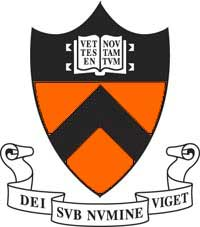# Allan SlyOffice: 405 Fine Hall
Email: asly@princeton.edu

Fine Hall
Department of Mathematics
Princeton NJ 08540

I am the Anthony H. P. Lee '79 Professor of Mathematics in the Department of Mathematics at Princeton University. I completed my PhD in Statistics at UC Berkeley in 2009 under the supervision of Elchanan Mossel and was then a postdoc in the Theory Group at Microsoft Research.

In Spring 2023 I am teaching MAT 589 on First and Last Passage Percolation (see canvas for details).

Previous Classes: Spring 2017 MAT 589, Fall 2017 MAT 385, Spring 2018 MAT 589, Fall 2019 MAT 486, Spring 2020 MAT 589.## Research

My research is in discrete probability theory and its applications to problems from statistical physics, theoretical computer science and theoretical statistics. Most of my work is centered on stochastic processes on networks in a range of different setting. Two major focuses are the analysis of the mixing times of Markov chains, particularly the Glauber dynamics and the role phase transitions play in the computational complexity and in probabilistic models more generally.

## Selected Works

A complete list of publications and preprints is available on Google Scholar.

Glauber Dynamics for the Ising Model
Cutoff for the Ising model on the lattice (with E. Lubetzky) Inventiones mathematicae, 191 (2013) 719--755. Arxiv
Critical Ising on the square lattice mixes in polynomial time (with E. Lubetzky) Communications in Mathematical Physics 313 (2013) 815--836. Arxiv

In these two papers we study the Glauber Dynamics Markov chain on the lattice. In two dimensions at high temperatures we established the cutoff phenomena a sharp transition of the dynamics from unmixed to mixed over a much smaller window of time than the mixing time. Recently, using a new tool we developed called information percolation, we have extended cutoff to the high temperature regime in all dimensions and at high enough temperatures on any graph. At the critical temperature the mixing time is expected to undergo a critical slowdown and become polynomial in the size of the system. We established a polynomial upper bound for the 2D Ising model combining multi-scale techniques for the Markov chains of spin systems with new results from the world of SLE.

Random Constraint Satisfaction Problems
Proof of the satisfiability conjecture for large k (with J. Ding and N. Sun) Proceedings of the 47th ACM Symposium on Theory of Computing (STOC) (2015) 59-68. Arxiv

Predictions from statistical physics give precise estimates of the satisfiability thresholds in a broad class of random constraint satisfaction problems based on relica symmetry breaking arguments. With Ding and Sun we rigorously established the satisfiability threshold for random k-SAT when k is sufficiently large at the threshold predicted by physicists. In related work we established the size of the largest independent set of a random regular graph showing that it has constant order fluctuations.

Computational Phase Transitions
Computational Transition at the Uniqueness Threshold Proceedings of IEEE Symposium on Foundations of Computer, 287-296 (2010). Co-winner of the best paper award. Arxiv.

This result, combined with work of Dror Weitz, gave the first example showing a computational threshold that is determined by the phase transition of a statistical physics model. Specifically we showed that in it is NP-hard to sample from the hardcore model on d-regular graphs when there is non-uniqueness for d-regular tree (and it is close enough to the threshold). One consequence is that it is NP-hard to approximately count independent sets on 6-regular graphs. With Nike Sun we extended this work to all anti-ferromagnetic two-spin systems. Together with results of Jerrum--Sinclair, Weitz, and Sinclair--Srivastava--Thurley, this gives an almost complete classification of the computational complexity of approximating the partition function in two-spin systems on bounded-degree graphs.

The Sparse Stochastic Block Model
A Proof Of The Block Model Threshold Conjecture (with E. Mossel and J. Neeman) To appear in Combinatorica. Arxiv

The stochastic block model is a classical random graph model containing a community structure. A conjecture of Decelle, Krzkala, Moore and Zdeborova based on ideas from statistical physics, gave a precise prediction for the threshold at which it is possible to recover (approximately) the clusters in the sparse stochastic block model. They conjectured that it corresponded to a spatial mixing threshold for the Ising model on a tree, the reconstruction threshold. In a series of work with Mossel and Neeman we established this conjecture finding efficient algorithms which succeed up to the predicted threshold. We also determined the optimal possible clustering and the point at which exact recovery of the clusters is possible.

The Slow Bond Problem
Last Passage Percolation with a Defect Line and the Solution of the Slow Bond Problem (with R. Basu and Vladas Sidoravicius) Submitted. Arxiv

The one dimensional totally asymmetric simple exclusion process (TASEP) is exactly solvable in the KPZ universality class and has been extensively studied with the current and its fluctuations well understood using powerful algebraic tools. When the rate of a single bond is perturbed, however, these methods are no longer applicable. In TASEP with step initial conditions, Janowsky and Lebowitz asked if any reduction in the jump rate of a bond was enough to reduce the long run asymptotic rate at which particles cross the origin. In this paper we found a more geometric approach establishing the conjecture.